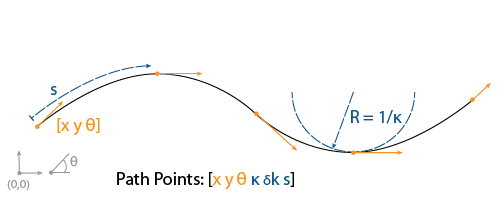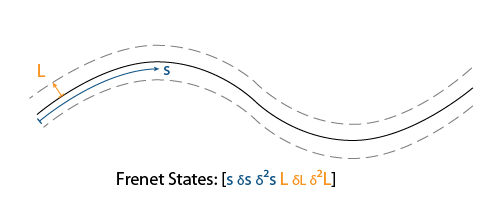referencePathFrenet

Smooth reference path fit to waypoints

Description

The referencePathFrenet object fits a smooth, piecewise, continuous curve to a set of waypoints given as [x y] or [x y theta]. After fitting, points along the curve, the path points are expressed as [x y theta kappa dkappa s], where:

• x y and theta— SE(2) state expressed in global coordinates, with x and y in meters and theta in radians

• kappa — Curvature, or inverse of the radius, in meters

• dkappa — Derivative of curvature with respect to arc length in meters per second

• s — Arc length, or distance along path from path origin, in metersUsing this object, convert trajectories between global and Frenet coordinate systems, interpolate states along the path based on arc length, and query the closest point on a path from a global state. The absolute difference between theta and the orientation of the closest point on the path from a global state must not exceed pi/2 when converting between global and Frenet coordinate systems.

The object expresses Frenet states as a vector of form [S dS ddS L dL ddL], where S is the arc length and L is the perpendicular deviation from the direction of the reference path. Derivatives of S are relative to time. Derivatives of L are relative to the arc length, S.Creation

Description

example

refPathObj = referencePathFrenet(waypoints) fits a piecewise, continuous set of curves to the specified waypoints. The waypoints argument sets the Waypoints property.

refPathObj = referencePathFrenet(waypoints,'DiscretizationDistance',discretionDist) fits a piecewise, continuous set of curves to waypoints using the specified distance between interpolated path points. The discretionDist argument sets the DiscretizationDistance property.

Properties

expand all

Arc length between interpolated path points, specified as a positive scalar in meters. The object uses interpolated path points to accelerate performance of the transformation functions frenet2global and global2frenet. A smaller discretization distance can improve accuracy at the expense of memory and computational efficiency.

Data Types: single | double

Presampled points along the path, specified as a P-by-2 matrix with rows of form [x y] or P-by-3 matrix with rows of form [x y theta]. Specify x and y in meters and theta in radians. P is the number of presampled points, and must be greater than or equal to two

Data Types: single | double

Object Functions

 closestPoint Find closest point on reference path to global point frenet2global Convert Frenet states to global states global2frenet Convert global states to Frenet states interpolate Interpolate reference path at provided arc lengths show Display reference path in figure

Examples

collapse all

Generate alternative trajectories for a reference path using Frenet coordinates. Specify different initial and terminal states for your trajectories. Tune your states based on the generated trajectories.

Generate a reference path from a set of waypoints. Create a trajectoryGeneratorFrenet object from the reference path.

waypoints = [0 0; ...
50 20; ...
100 0; ...
150 10];
refPath = referencePathFrenet(waypoints);
connector = trajectoryGeneratorFrenet(refPath);

Generate a five-second trajectory between the path origin and a point 30 m down the path as Frenet states.

initState = [0 0 0 0 0 0];  % [S ds ddS L dL ddL]
termState = [30 0 0 0 0 0]; % [S ds ddS L dL ddL]
[~,trajGlobal] = connect(connector,initState,termState,5);

Display the trajectory in global coordinates.

show(refPath);
hold on
axis equal
plot(trajGlobal.Trajectory(:,1),trajGlobal.Trajectory(:,2),'b')
legend(["Waypoints","Reference Path","Trajectory to 30m"])Create a matrix of terminal states with lateral deviations between –3 m and 3 m. Generate trajectories that cover the same arc length in 10 seconds, but deviate laterally from the reference path. Display the new alternative paths.

termStateDeviated = termState + ([-3:3]' * [0 0 0 1 0 0]);
[~,trajGlobal] = connect(connector,initState,termStateDeviated,10);

clf
show(refPath);
hold on
axis equal
for i = 1:length(trajGlobal)
plot(trajGlobal(i).Trajectory(:,1),trajGlobal(i).Trajectory(:,2),'g')
end
legend(["Waypoints","Reference Path","Alternative Trajectories"])
hold offSpecify a new terminal state to generate a new trajectory. This trajectory is not desirable because it requires reverse motion to achieve a lateral velocity of 10 m/s.

newTermState = [5 10 0 5 0 0];
[~,newTrajGlobal] = connect(connector,initState,newTermState,3);

clf
show(refPath);
hold on
axis equal
plot(newTrajGlobal.Trajectory(:,1),newTrajGlobal.Trajectory(:,2),'b');
legend(["Waypoint","Reference Path","New Trajectory"])
hold offRelax the restriction on the longitudinal state by specifying an arc length of NaN. Generate and display the trajectory again. The new position shows a good alternative trajectory that deviates off the reference path.

relaxedTermState = [NaN 10 0 5 0 0];
[~,trajGlobalRelaxed] = connect(connector,initState,relaxedTermState,3);

clf
show(refPath);
hold on
axis equal
plot(trajGlobalRelaxed.Trajectory(:,1),trajGlobalRelaxed.Trajectory(:,2),'g');
hold off